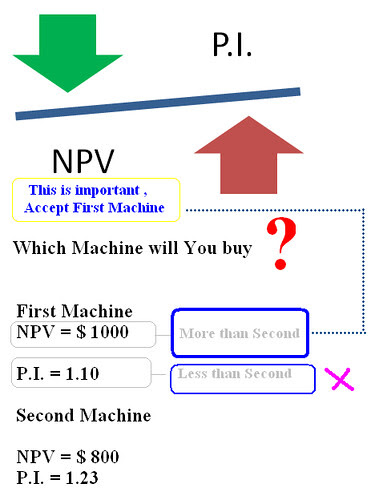Profitability Index has made his identity as the technique of evaluation of investment projects in financial market. This is fresh method and if any body has learnt NPV of capital budgeting, then he can also understand profitability index. In profitability Index, we also calculate the present value of cash outflows or investments for purchasing projects or assets and present value of cash inflows in the form of profit from investments. We use discount or minimum rate for calculating present value. After this profitability Index is calculated by dividing PV of cash inflows with PV of cash outflows.

P.I. = Present Value of Cash inflows / Present Value of Cash outflows

Profitability Index shows profitability, if we invest per unit of money. On the basis of profitability index, we can provide ranks to different investment projects. Higher P.I. will get higher rank and lower P.I. will get lower rank and only highest rank project will be accepted for real investment. If there is only one project and we have to decide whether investment in this project is profitable or not. This decision can be taken by calculating profitability index. If profitability index is one or more, then project should be accepted. A ratio of 1.0 is logically the lowest acceptable measure on the index. Any value lower than 1.0 would indicate that the project's PV is less than the initial investment. As values on the profitability index increase, so does the financial attractiveness of the proposed project.

NPV Vs Profitability Index

NPV method and profitability index are deeply connected with each other and both give same result for evaluation of investment projects. We accept that investment proposal whose NPV must be positive and we also accept that investment proposal whose P.I. must be more than one. If  we compare two projects by applying both NPV and Profitability index and if NPV of first project is more than second project but Profitability index is less than second, at time, we will prefer first project because NPV will be capable to maximize the fund of shareholders..

## \$type=three\$a=hide\$cm=hide\$s=hide\$show=/2019/06/10-steps-to-become-better-investor.html\$l=hide

Name

false
ltr
item
Accounting Education: Profitability Index
Profitability Index
http://farm5.static.flickr.com/4036/4420395102_5a894d3b5c.jpg
http://farm5.static.flickr.com/4036/4420395102_5a894d3b5c_t.jpg
Accounting Education
https://www.svtuition.org/2010/03/profitability-index.html
https://www.svtuition.org/
http://www.svtuition.org/
http://www.svtuition.org/2010/03/profitability-index.html
true
2410664366776677676
UTF-8